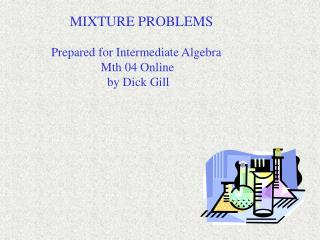DownloadDownload PresentationMIXTURE PROBLEMS

# MIXTURE PROBLEMS

Download Presentation## MIXTURE PROBLEMS

- - - - - - - - - - - - - - - - - - - - - - - - - - - E N D - - - - - - - - - - - - - - - - - - - - - - - - - - -
##### Presentation Transcript

1. MIXTURE PROBLEMS Prepared for Intermediate Algebra Mth 04 Online by Dick Gill

2. The following slides give you nine mixture problems to practice. Answers to these problems follow. If some of your answers are wrong, the complete solutions will follow the answer slide.

3. Three mixture problems at the beginners level. Round your answers • to the nearest tenth if necessary. • How many ounces of a solution that is 10% alcohol should be • mixed with 12 ounces of a solution that is is 24% alcohol to create • a solution that is 15% alcohol? • How many liters of a solution that is 20% acid should be added • to 3 liters of a solution that is 30% acid to create a solution that is • 24% acid? • How many liters of a solution that is 50% antifreeze should be • added to 8 liters of a solution that is 80% antifreeze to create a • solution that is 60% antifreeze?

4. Three mixture problems at the intermediate level. Round your answers to the nearest tenth if necessary. 4. How many ounces of a solution that is 10% alcohol should be added to a solution that is 28% alcohol to create 30 ounces of a solution that is 20% alcohol? 5. How many liters of a solution that is 20% acid should be added to how many liters of a solution that is 38% acid to create 8 liters of a solution that is 30% acid? 6. How many liters of a solution that is 50% antifreeze should be added to how many liters of a solution that is 72% antifreeze to create 2.4 liters of a solution that is 58% antifreeze?

5. Three mixture problems at the advanced level. Round your answers to the nearest hundredth if necessary. 7. Ten liters of a solution that is 30% alcohol is going to be diluted to 24% alcohol by adding water. How much water is needed? 8. A solution that is 30% antifreeze is going to be enriched by adding pure antifreeze. How much of each is needed to generate 2 gallons of a solution that is 50% antifreeze? 9. How many gallons should be drained from a 10 gallon tank of 24% alcohol if we are going to replace it with pure alcohol and create a solution of 35% alcohol?

6. Answers to mixture problems 1 – 9. • 21.6 ounces • 4.5 liters • 16 liters • 13.3 ounces • 3.6 liters at 20%; 4.4 liters at 38% • 1.5 liters at 50%; 0.9 liters at 72% • 2.5 liters • 1.43 gal at 30%; 0.57 gal at 100% • 1.45 gal • Complete solutions follow.

7. 1. .10x + .24(12) = .15(x + 12) .10x + 2.88 = .15x + 1.8 1.08 = .05x 21.6 = x 21.6 ounces @ 10%

8. 2. .20x + .90 = .24x + .72 .18 = .04x 4.5 = x 4.5 liters at 20%

9. 3. .50x + .80(8) = .60(x + 8) .50x + 6.40 = .60x + 4.80 1.60 = .10x 16 = x 16 liters at 50%

10. 4. .10x + .28(30 – x) = .20(30) .10x + 8.4 - .28x = 6 -.18x = -2.4 x = 13.3 13.3 ounces at 10%

11. 5. .20x + .38(8 – x) = .30(8) .20x + 3.04 - .38x = 2.40 -.18x = -.64 x = 3.6; 8 – x = 4.4 3.6 liters at 20%; 4.4 liters at 38%

12. 6. .50x + .72(2.4 – x) = .58(2.4) .50x + 1.728 - .72x = 1.392 -.22x = -.336 x = 1.5; 2.4 – x = 0.9 1.5 liters at 50%; 0.9 liters at 72%

13. 7. .30(10) + 0 = .24(x + 10) 3 = .24x + 2.4 0.6 = .24x 2.5 = x 2.5 liters of water

14. 8. .30(x) + 1.00(2 – x) = .50(2) .30x + 2.00 – 1.00x = 1.00 -.70x = -1.00 x = 1.43; 2 – x = 0.57 1.43 gal at 30%; 0.57 gal at 100%

15. 9. .24(10 – x) + 1.00x = .35(10) 2.4 - .24x + 1.00x = 3.5 .76x = 1.1 x = 1.45 Drain 1.45 gal and replace with 100% alcohol